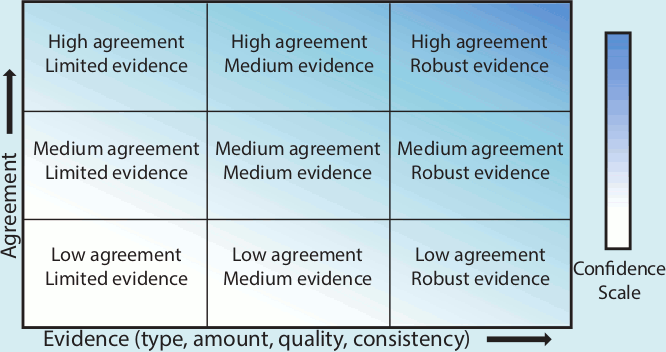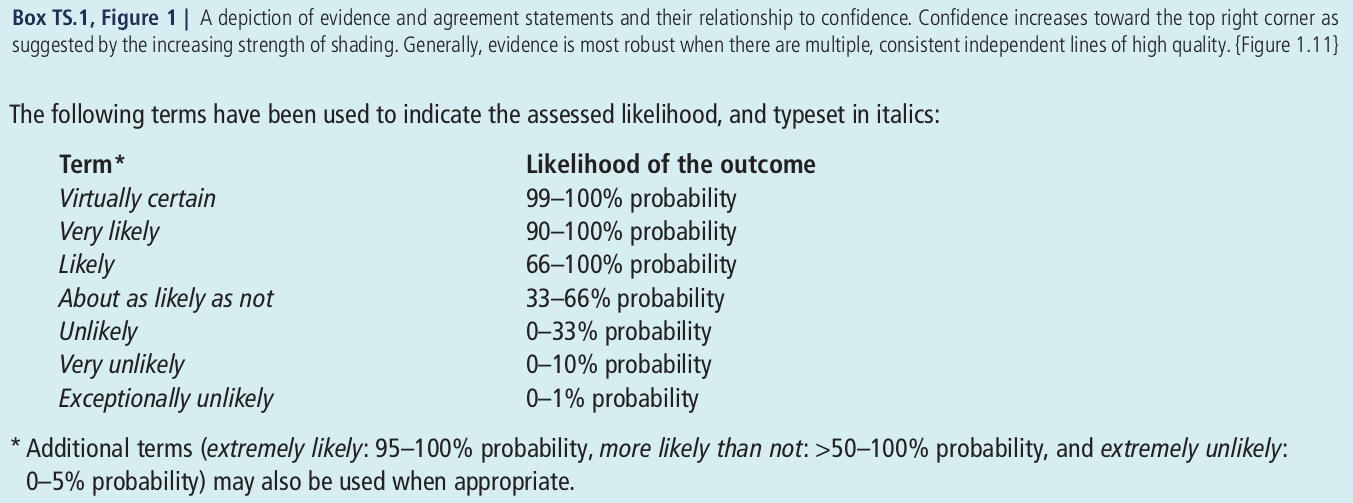﻿ Expression of uncertainty
Climate change (Class of 2019)

Expression of uncertainty (ja:不たしかさ、不確実性) by IPCC

Since the 3rd assessment report (2001), IPCC tries to express how uncertain their pieces of knowledge are. Since the 4th assessment report (2007), they use two technical terms, "likelihood" and "confidence". Unfortunately, here is a possible source of confusion because of "expert community dialects".

"Likelihood" (ja:可能性) in IPCC is a crude measure of probability (ja:確率). (Not "likelihood" (ja:尤度[ゆうど]) in mathematical statistics.) I prefer wording with "probability" even if numerical values of probabilty are not mentioned.

"Confidence" (ja:確信度) in IPCC means how well the piece of knowledge is supported by evidence. (Not "confidence (interval)" (ja:信頼 (区間)) in mathematical statistics.) I prefer "robustness (of knowledge)" (ja:(知識の)頑健性), following philosophers of science. (Not "robustness" (of methods of estimation) in mathematical statistics, either.)

The following explanation is from IPCC 5th Assessment Report, Working Group 1 (2013), Technical Summary, Box 1.2019-June-20
MASUDA Kooiti